• 由于概率和期望具有线性性质，使得可以在概率和期望之间建立一定的递推关系，这样就可以通过动态规划来解决一些概率问题，例如概率期望的最值问题就常常使用概率 DP、期望 DP 来解决。 其他的动态规划一样，...
【概述】

由于概率和期望具有线性性质，使得可以在概率和期望之间建立一定的递推关系，这样就可以通过动态规划来解决一些概率问题，例如概率和期望的最值问题就常常使用概率 DP、期望 DP 来解决。

与其他的动态规划一样，合理的选择状态以及高效的状态转移方程是关键，选择合适的状态不仅可以提高效率，而且可以保证动态规划所必须的无后效性。

一般来说，将问题直接作为状态是最好的，当找到正确的状态定义后，转移是较容易想到的，一般的递推即可确定转移。

例如：n 个人做 XX 的期望次数，可以设计状态 f[i] 表示 i 个人做完事的期望。

【概率 DP】

概率 DP 通常已知初始的状态，然后求解最终达到目标的概率，因此概率 DP 需要顺序求解。

其相较于期望 DP较为简单，当前状态只需加上所有上一状态乘上转移概率即可，即：f[i]=f[i-1]*p[i]

【期望 DP】

期望 DP 与概率 DP 不同，其需要倒序求解。

当求解达到某一目标的期望花费时，由于最终的花费无从知晓（无法从无穷推起），因此期望 DP 需要倒序求解。

设 f[i] 为 i 状态下实现目标的期望值，即到了 f[i] 这个状态的差距是多少。

初始时，令 f[n]=0，即在目标状态期望值为 0，然后进行状态转移，新的状态为上一状态与转移概率的乘积再加上转移的花费

即：f[i-1]=f[i]*p[i]+w

最后初始位置 f 即为所求的期望值

需要注意的是，当转移关系不成环时，期望 DP 可以进行线性递推，但当转移关系成环时，期望 DP 的最终状态相当于一个已知量，而转移关系相当于一个个方程，此时需要使用高斯消元法来解决。

【例题】

Discovering Gold（LightOJ-1030）(期望DP)：点击这里
Dice (III)（LightOJ-1248）(期望DP)：点击这里
Just another Robbery（LightOJ-1079）(概率DP+01背包)：点击这里
Let's Play Osu!（CF-236D）(期望DP)：点击这里
Piglet's Birthday（CF-248E）(概率DP)：点击这里
Robots（2019 ACM-ICPC 南京赛区网络赛 D）(期望DP)：点击这里
Everything Is Generated In Equal Probability（HDU-6595）(期望DP)：点击这里
Kejin Player（HDU-6656）(期望DP+降维)：点击这里

展开全文• 我们可以倒推，从后向前转移，因为后面状态合法前面状态是否合法是没有关系的。倒推也是规避不合法状态一种有效手段。这样最后结果就是f。 我表示不知道期望是什么东西。。更不知道怎么算。。膜...
我们先考虑dp。
n很小，并且状态之间有明显的依赖关系，显然可以二进制状压。但是为了保证当前状态是从一个合法的状态转移而来是不好判断的。我们可以倒推，从后向前转移，因为后面的状态合法与前面的状态是否合法是没有关系的。倒推也是规避不合法状态的一种有效手段。这样最后的结果就是f。
我表示不知道期望是什么东西。。更不知道怎么算。。膜一发题解吧QAQ。

这一步的期望=(上一步的期望+这一步的得分)/K。

#include<iostream>
#include<cstdio>
using namespace std;
double f[1<<15];
int n,K,v,d;
inline int read()
{
int a=0,f=1; char c=getchar();
while (c<'0'||c>'9') {if (c=='-') f=-1; c=getchar();}
while (c>='0'&&c<='9') {a=a*10+c-'0'; c=getchar();}
return a*f;
}
int main()
{
K=read(); n=read();
for (int i=1;i<=n;i++)
{
v[i]=read();
int s=read();
while (s) d[i]|=1<<(s-1),s=read();
}
for (int i=K;i;i--)
for (int j=0;j<(1<<n);j++)
{
for (int k=1;k<=n;k++)
if ((d[k]&j)==d[k])
f[i][j]+=max(f[i+1][j],f[i+1][j|(1<<(k-1))]+v[k]);
else f[i][j]+=f[i+1][j];
f[i][j]/=n;
}
printf("%.6lf",f);
return 0;
}
展开全文• 随机变量(Random Variable)X是一个映射，把随机试验的结果实数建立起了一一对应的关系。而期望与方差是随机变量的两个重要的数字特这。 期望(Expectation, or expected value)是度量一个随机变量取值的集中位置或...
随机变量(Random Variable)X是一个映射，把随机试验的结果与实数建立起了一一对应的关系。而期望与方差是随机变量的两个重要的数字特这。
期望(Expectation, or expected value)是度量一个随机变量取值的集中位置或平均水平的最基本的数字特征；
方差(Variance)是表示随机变量取值的分散性的一个数字特征。 方差越大，说明随机变量的取值分布越不均匀，变化性越强；方差越小，说明随机变量的取值越趋近于均值，即期望值。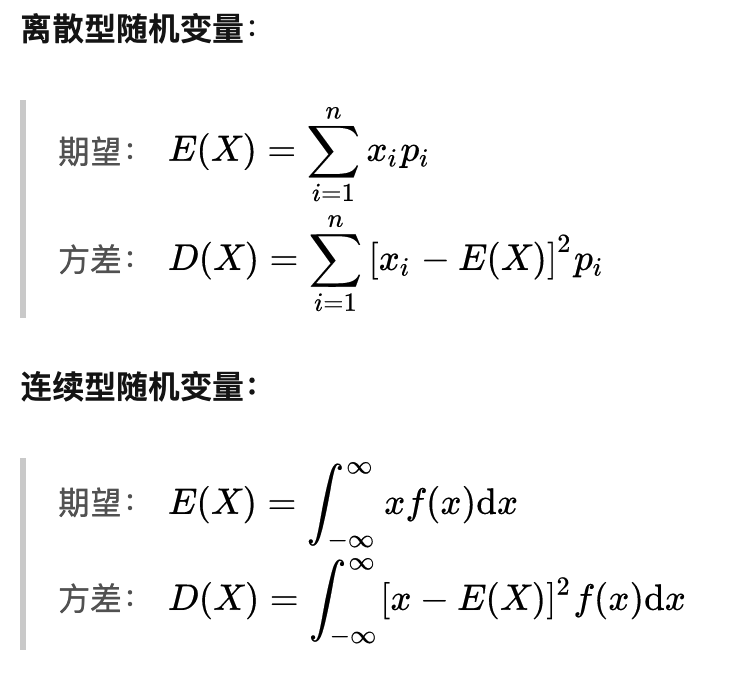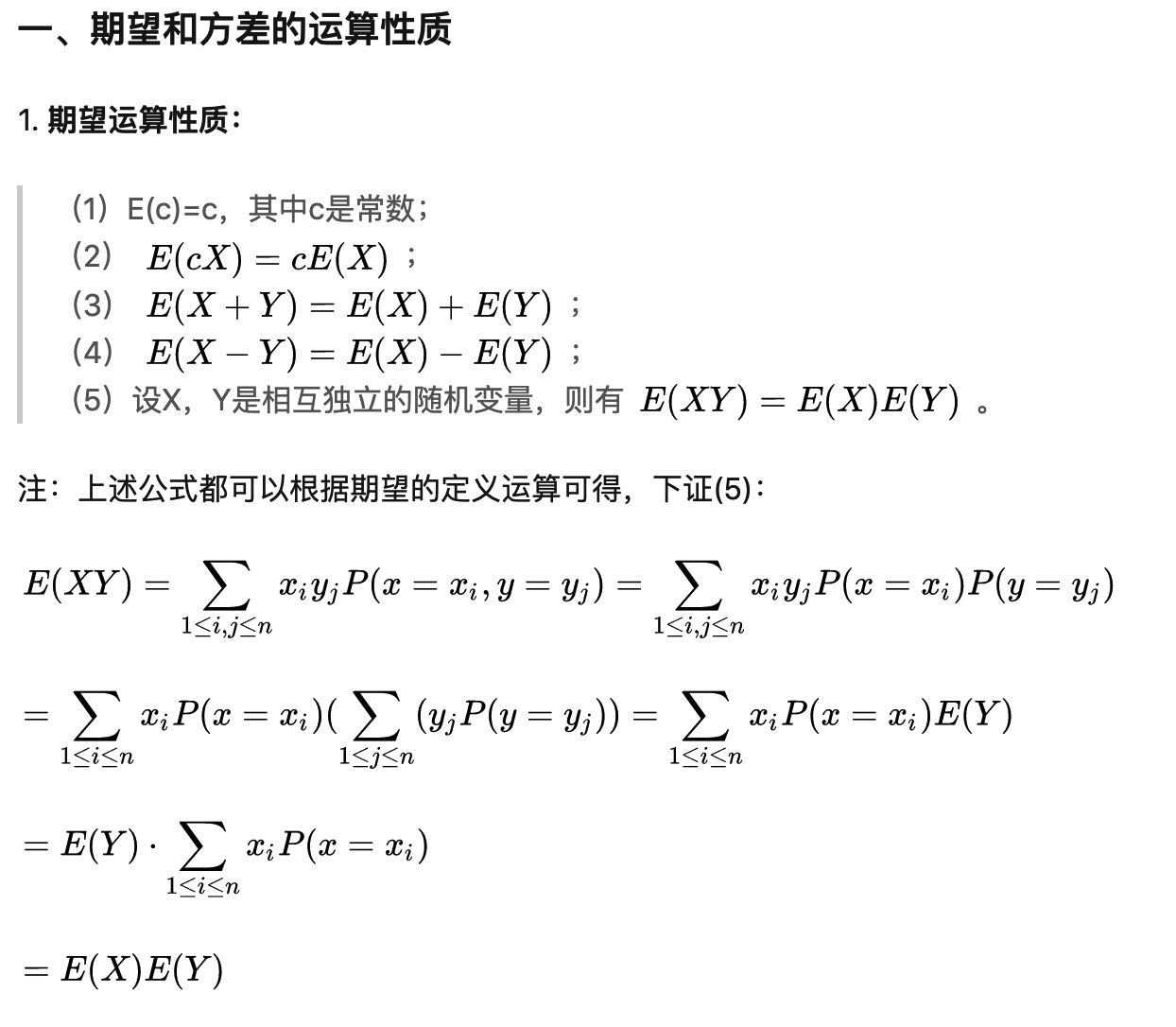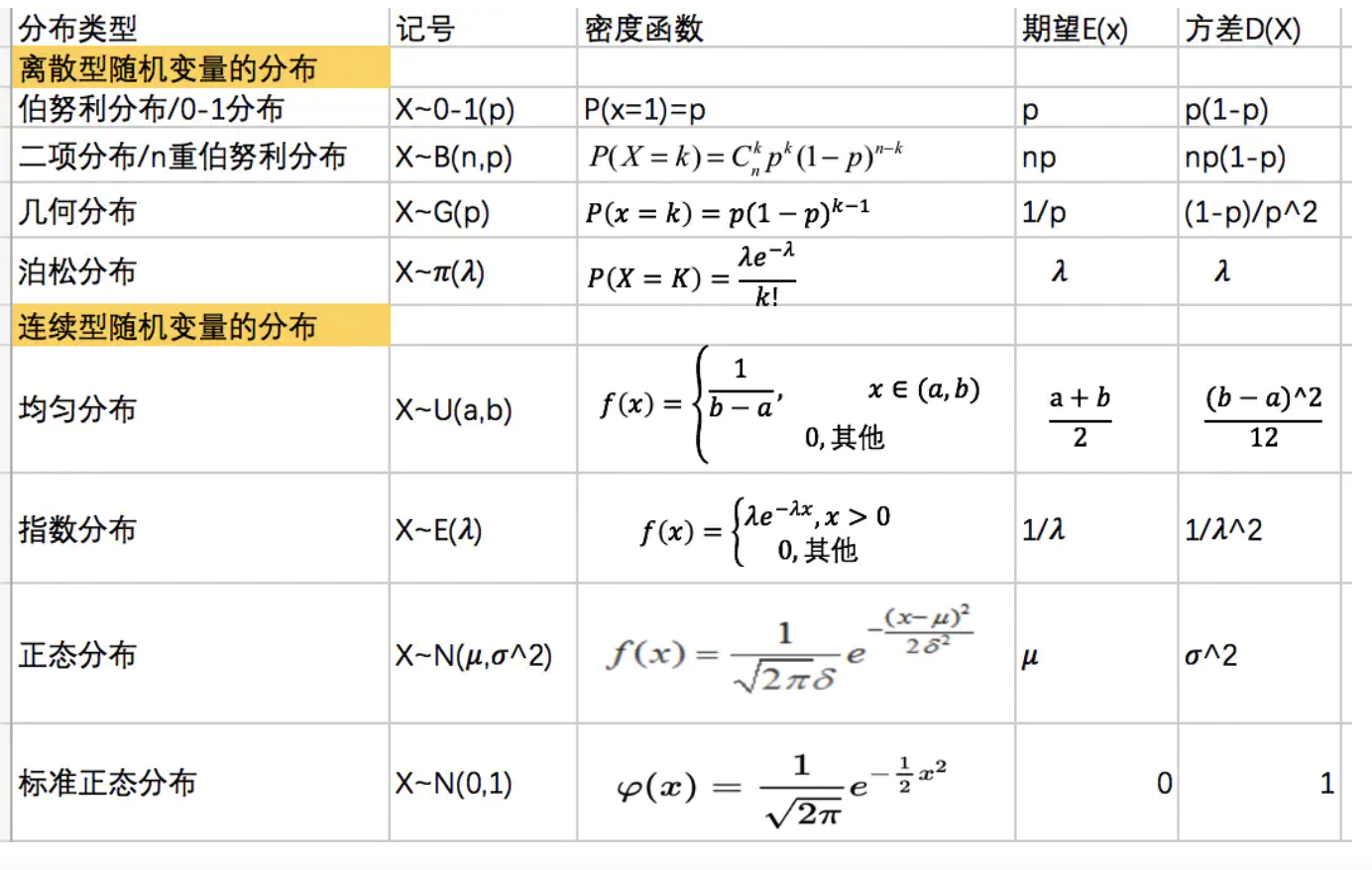贝叶斯例题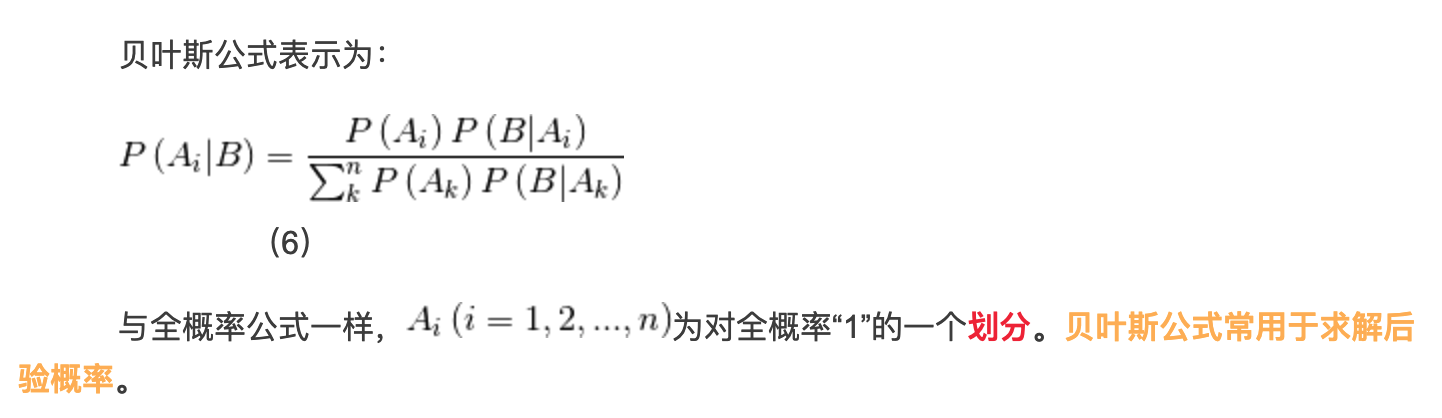简单理解一下就是： 当B发生的前提下，求Ai 事件发生的概率，
但是可以看出，当B发生时，其实A事件的 全概率划分(A1,A2,Ai)都会发生，我们需要找到 当B发生时，Ai 事件发生概率 在 全概率事件中的 可能性(概率)， 既
P (Ai发生的概率 | 当B发生时 )=  Ai发生的概率 $\times$ Ai发生时B发生的概率$\div$ 求和k（Ak发生的概率 $\times$ Ak发生时B发生的概率）。
理解一下全概率事件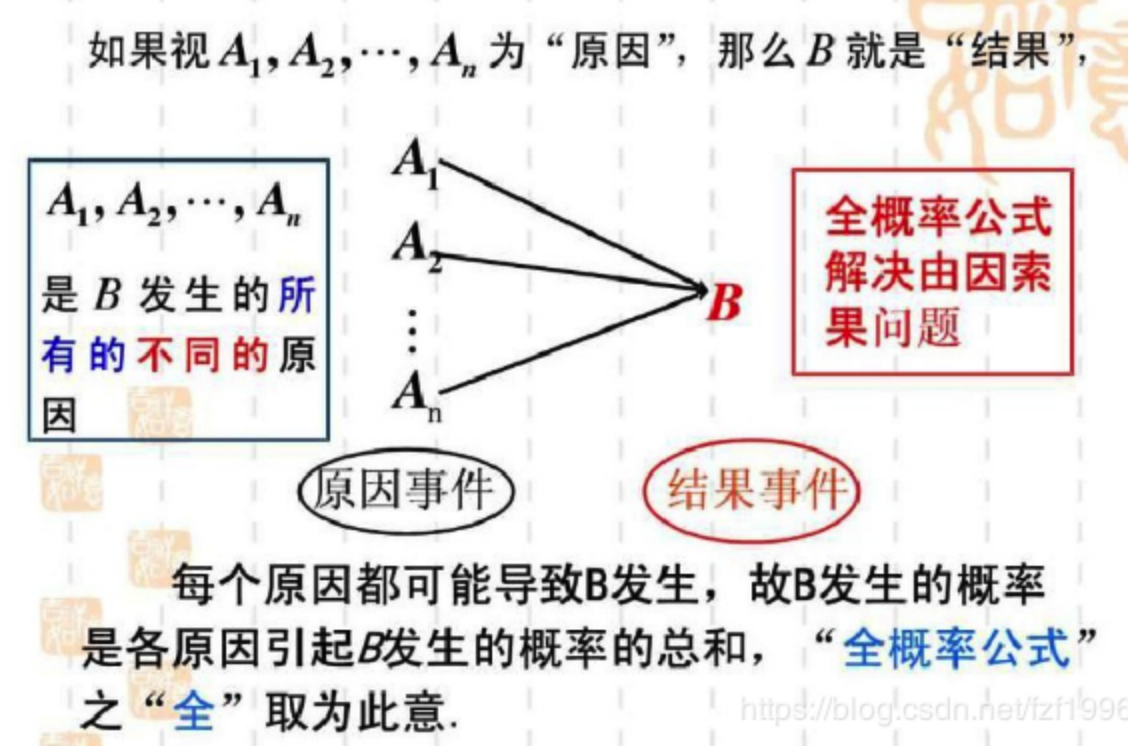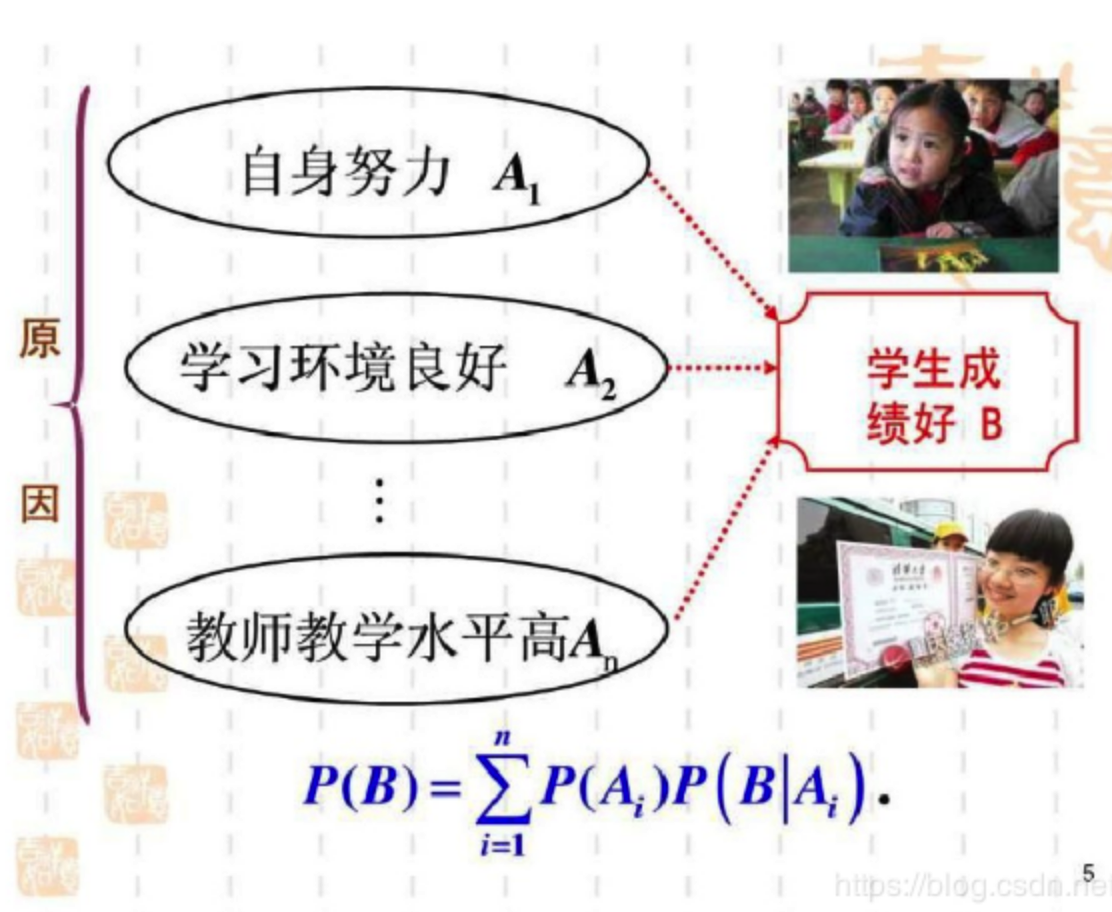就如我们上面提到的，在B发生前提下，找A事件发生的概率， 既在 所有造成B事件的 原因中(全概率事件)，A事件发生的 概率；
P(A|B) = P(A,B) $\div$ P(B) = P(B|A) $\times$ P(A) $\div$ P(B)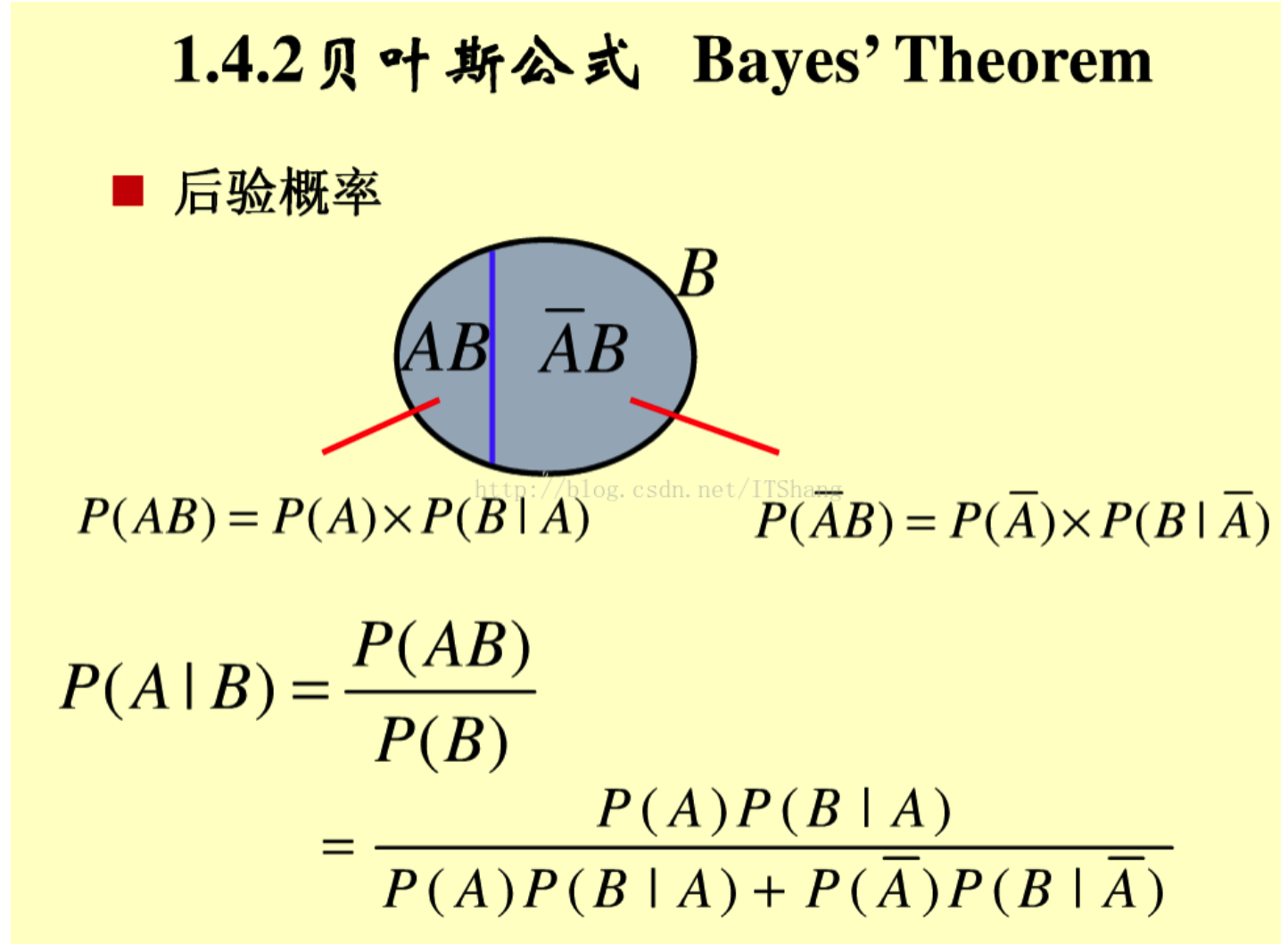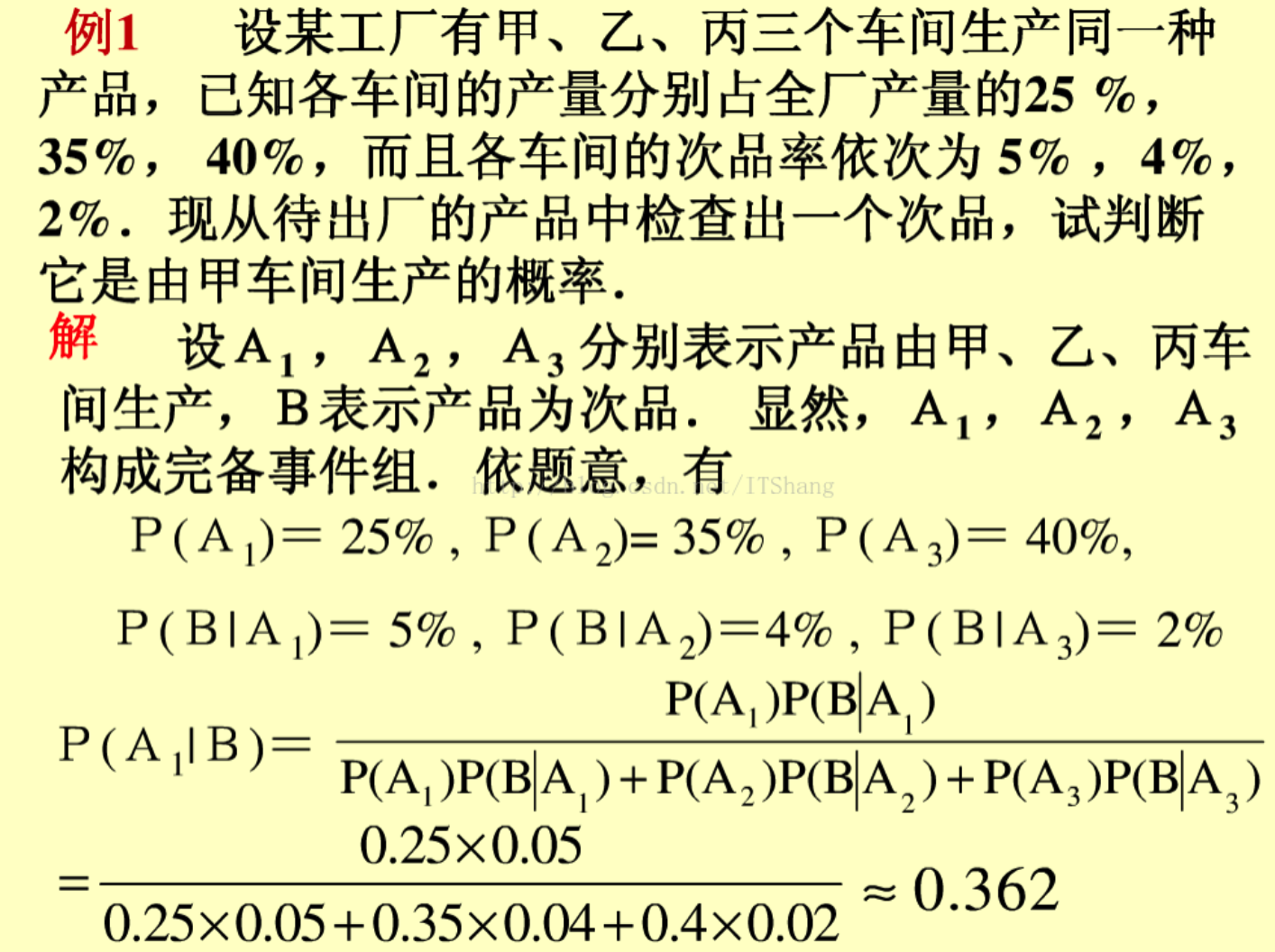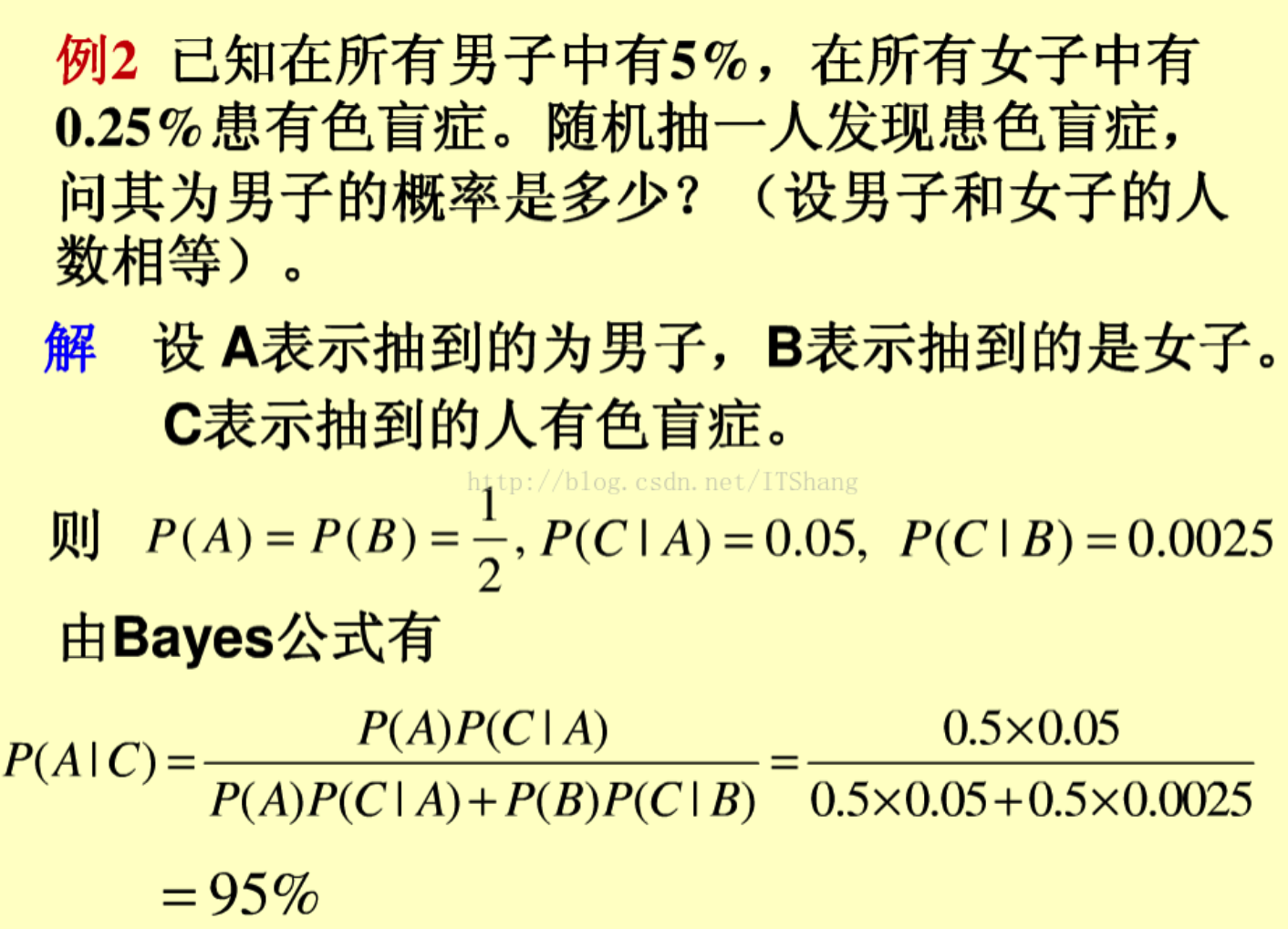4. 信号塔问题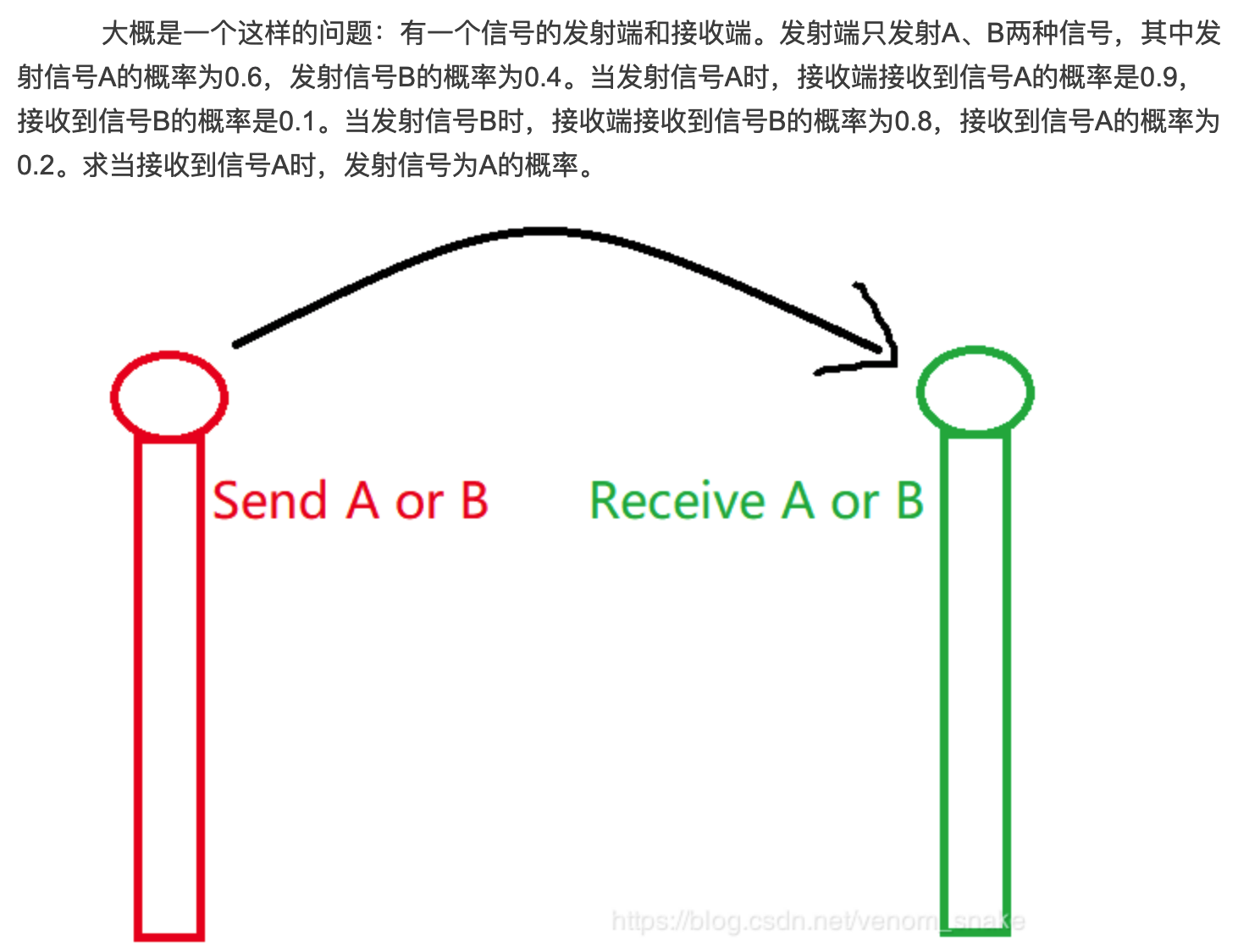求解 P( SA | RA) = ？
既在 已知接收A信号前提下，  求发射A 的概率？
我们可知 P(SA) = 0.6  P(!SA) = 0.4
根据全概率和贝叶斯公式： P(SA|RA) = P(SA,RA) / P(RA)
P(SA|RA) = P(RA|SA).P(SA) / P(RA)
其中发生接受A信号这个事件，  可能从不同的 SA 子事件造成，如 发射A接受A, 发射B接受A ,  我们需要 统计所有 发生 接受A 的事件概率，  并从这个事件集合中，找到 目标状态(发射A) 的占比概率；
患病类的贝叶斯题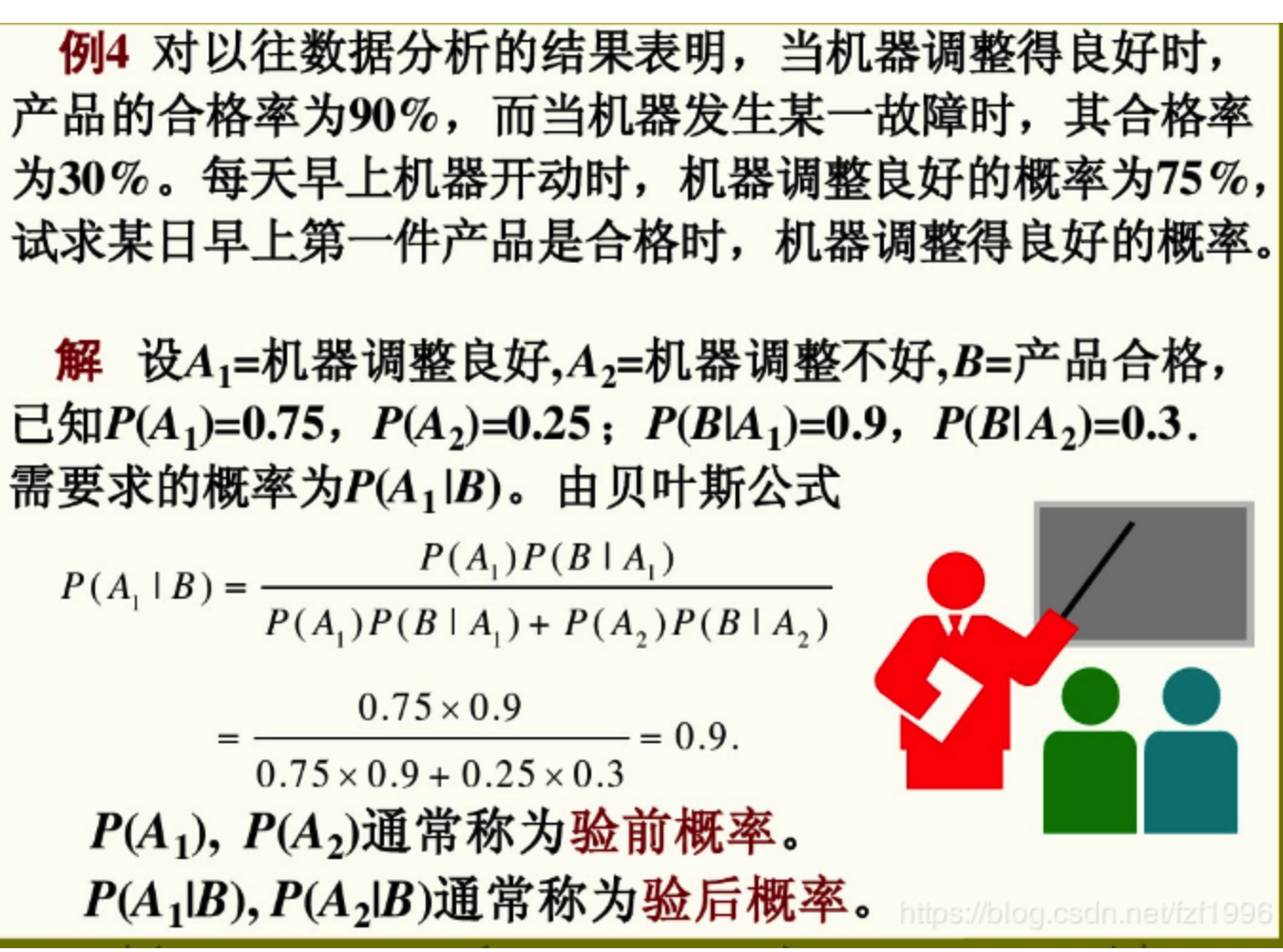关于概率类的例题总结的很棒
有8个箱子，现在有一封信，这封信放在这8个箱子中（任意一个）的概率为4/5,不放的概率为1/5（比如忘记了）,现在我打开1号箱子发现是空的，求下面7个箱子中含有这封信的概率为？
求 已知1号箱空的前提条件， 信出现在 其它7个箱子的 概率；
P(箱中 | 1号空)  = P(箱中，1号空) / P(1号空）
P(1号空) 的全概率事件为 = P(1号空|箱中)P(箱中） + P(1号空|非箱中)P(非箱中)
= 7/8 * 4/5 + 1 * 1/5
牛客整理的概率题


展开全文• 根据期望的线性性，我们只需对于每两个位置(i,j)(i,j)(i,j)计算出其相对位置改变的概率，并根据aiaia_i和ajaja_j的大小关系统计贡献即可。 于是我们不难得到一个O(n2k)O(n2k)O(n^2k)的DP。设fi,j,kfi,j,kf_{i,j,k}...
根据期望的线性性，我们只需对于每两个位置(i,j)$\left(i,j\right)$$(i,j)$计算出其相对位置改变的概率，并根据ai${a}_{i}$$a_i$和aj${a}_{j}$$a_j$的大小关系统计贡献即可。

于是我们不难得到一个O(n2k)$O\left({n}^{2}k\right)$$O(n^2k)$的DP。设fi,j,k${f}_{i,j,k}$$f_{i,j,k}$表示当前(i,j)$\left(i,j\right)$$(i,j)$两个位置再进行k$k$$k$步交换操作使得i>j$i>j$$i>j$的概率（边界就是fi,j,0=[i>j]${f}_{i,j,0}=\left[i>j\right]$$f_{i,j,0}=[i>j]$），显然可以从fi,j,k−1,fj,i,k−1,∑t≠jfi,t,k−1,∑t≠ift,j,k−1${f}_{i,j,k-1},{f}_{j,i,k-1},\sum _{t\ne j}{f}_{i,t,k-1},\sum _{t\ne i}{f}_{t,j,k-1}$$f_{i,j,k-1},f_{j,i,k-1},\sum_{t\neq j}f_{i,t,k-1},\sum_{t\neq i}f_{t,j,k-1}$乘上其对应的概率转移过来，后两个和式先处理出来就可以O(1)$O\left(1\right)$$O(1)$转移了。

考虑优化这个DP。加入我们把两个位置(i,j)$\left(i,j\right)$$(i,j)$看成一个去除了直线x=y$x=y$$x=y$二维平面上的一个点，那么问题就转化成对于x=y$x=y$$x=y$上方的每一个点，求出x=y$x=y$$x=y$下方的所有点转移k$k$$k$步之后能到达它的概率之和，其中转移分别是：
1. 不动，P=z−2n+3$P=z-2n+3$$P=z-2n+3$。
2. 移动到当前行的另一个点，P=n−2$P=n-2$$P=n-2$。
3. 移动到当前列的另一个点，P=n−2$P=n-2$$P=n-2$。
4. 移动到关于x=y$x=y$$x=y$的对称点，P=1$P=1$$P=1$。

其中z=n(n−1)2$z=\frac{n\left(n-1\right)}{2}$$z=\frac{n(n-1)}{2}$。通过观察发现，平面上所有点经过若干次操作走到给定点(i,j)$\left(i,j\right)$$(i,j)$的概率只有本质不同的5$5$$5$种。具体如下：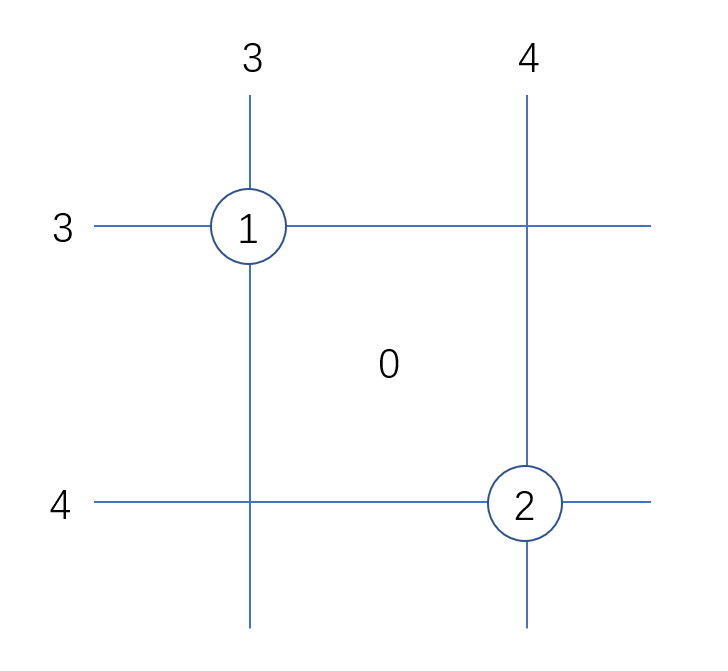其中1$1$$1$是给定点(i,j)$\left(i,j\right)$$(i,j)$，2$2$$2$为其对称点，3$3$$3$为与(i,j)$\left(i,j\right)$$(i,j)$同行同列的其它点，4$4$$4$为与(i,j)$\left(i,j\right)$$(i,j)$同行同列的其它点，0$0$$0$为除掉以上四种剩下的点。注意到3,4$3,4$$3,4$之间其实是没有交的，因为直线x=y$x=y$$x=y$已经被除去了。
那么这5$5$$5$种情况相互之间的转移矩阵就是：
⎡⎣⎢⎢⎢⎢⎢⎢z−400n−3n−30z−2n+311001z−2n+30122n−40z−n2202n−42z−n⎤⎦⎥⎥⎥⎥⎥⎥$\left[\begin{array}{ccccc}z-4& 0& 0& 2& 2\\ 0& z-2n+3& 1& 2n-4& 0\\ 0& 1& z-2n+3& 0& 2n-4\\ n-3& 1& 0& z-n& 2\\ n-3& 0& 1& 2& z-n\end{array}\right]$
\left[
\begin{array}{ccccc}
z-4&0&0&2&2\\
0&z-2n+3&1&2n-4&0\\
0&1&z-2n+3&0&2n-4\\
n-3&1&0&z-n&2\\
n-3&0&1&2&z-n\\
\end{array}
\right]

矩阵快速幂搞出所有系数。有了系数我们就可以n2${n}^{2}$$n^2$枚举(i,j)$\left(i,j\right)$$(i,j)$，统计在x=y$x=y$$x=y$下方每种点的个数即可。但我们观察到：0$0$$0$有z−2n−3$z-2n-3$$z-2n-3$个；1$1$$1$有0$0$$0$个；2$2$$2$有1$1$$1$个；3$3$$3$有n−j+i−1$n-j+i-1$$n-j+i-1$个；4$4$$4$有n−i+j−3$n-i+j-3$$n-i+j-3$个。都只和j−i$j-i$$j-i$有关，于是我们可以用树状数组分别统计出顺序对/逆序对的对数和∑(j−i)$\sum \left(j-i\right)$$\sum(j-i)$，就可以一起算了。总复杂度O(nlogn+125logk)$O\left(n\mathrm{log}n+125\mathrm{log}k\right)$$O(n\log n+125\log k)$。

代码：

#include<iostream>
#include<cstdio>
#include<cstring>
#define ll long long
#define N 500010
#define up(x,y) (x=(x+(y))%mod)
using namespace std;
const int mod=998244353;
int n,K,a[N];
ll z,iz,ans,cnt;
int read()
{
int x=0,f=1;char ch=getchar();
for(;ch<'0'||ch>'9';ch=getchar()) if(ch=='-') f=-1;
for(;ch>='0'&&ch<='9';ch=getchar()) x=x*10+ch-'0';
return x*f;
}
ll ksm(ll a,ll b)
{
ll r=1;
for(;b;b>>=1,a=a*a%mod)
if(b&1) r=r*a%mod;
return r;
}
struct matrix
{
ll a;
matrix(){memset(a,0,sizeof(a));}
matrix operator *(matrix b)
{
matrix re;
for(int i=0;i<5;i++)
for(int j=0;j<5;j++)
for(int k=0;k<5;k++)
up(re.a[i][j],a[i][k]*b.a[k][j]);
return re;
}
void init()
{
a=z-4;        a=0;      a=0;      a=2;      a=2;
a=0;          a=z-2*n+3;a=1;      a=2*n-4;  a=0;
a=0;          a=1;      a=z-2*n+3;a=0;      a=2*n-4;
a=n-3;        a=1;      a=0;      a=z-n;    a=2;
a=n-3;        a=0;      a=1;      a=2;      a=z-n;
}
}T;
matrix matksm(matrix a,ll b)
{
matrix r;
for(int i=0;i<5;i++)
r.a[i][i]=1;
for(;b;b>>=1,a=a*a)
if(b&1) r=r*a;
return r;
}
struct bit
{
int c[N];
void add(int x,ll d)
{
for(;x<=n;x+=(x&-x))
up(c[x],d);
}
ll qry(int x)
{
ll r=0;
for(;x;x-=(x&-x))
up(r,c[x]);
return r;
}
}t1,t2;
ll cal(ll num,ll sum)
{
ll re=0;
cnt=0;cnt=num;cnt=(num*(n-1)-sum+mod)%mod;cnt=(num*(n-3)+sum)%mod;
cnt=((z*num-cnt-cnt-cnt-cnt)%mod+mod)%mod;
for(int k=0;k<5;k++)
up(re,T.a[k]*cnt[k]);
return re;
}
int main()
{
n=read();K=read();
for(int i=1;i<=n;i++)
a[i]=read();
z=((ll)n*(n-1)/2)%mod;
iz=ksm(z,mod-2);
T.init();
T=matksm(T,K);
ll cnt,num=0,sum=0,P=0;
for(int i=1;i<=n;i++)
{
ll tmp=t1.qry(a[i]);
up(num,tmp);
up(sum,tmp*i-t2.qry(a[i])%mod+mod);
t1.add(a[i],1);
t2.add(a[i],i);
}
up(ans,cal(num,sum));
num=z-num;sum=-sum;
for(int i=1;i<n;i++)
up(sum,(ll)i*(n-i));
up(ans,ksm(z,K)*num%mod-cal(num,sum)+mod);
printf("%lld",ans);
return 0;
}
展开全文• 怪物猎人脚本 该存储库包含源代码，该网站提供了怪物猎人相关计算器和工具。 它还包含可直接从... 这会给出来自不同来源物品详细期望值，包括任务奖励，雕刻，捕获和闪亮掉落。 它还提供了建议，使哪些任HTML
• 首先，用期望的线性性质，那么答案为所有点有电的概率和 发现一个点的有电的概率来源形成了一个"或"关系，在概率中，这并不好计算...（其实是可以算的，只不过式子要复杂点...） 考虑反面，一个点没电的概率来源是...
• 分析总结：通过这道树形dp，加深了对换根理解，对于解决一些有依赖性关系的问题，我们可以考虑高斯消元/换根dp，主要套路为先一遍dfs求出每个结点只考虑子节点中贡献，那么作为根，它答案就是和我们想求一致...
• 分析总结：显然要求每条边经过次数的期望，设边(u->x)的期望是exp(u−>x)exp(u->x)exp(u−>x),那么exp(u−>x)=exp(u)/d[u]+exp[x]/d[x]exp(u->x)=exp(u)/d[u]+exp[x]/d[x]exp(u−>x)
• 题目如下： 有一个六个面均匀骰子，...首先，如果在第n次失败了，那么第n+1次之后抛骰子行为是前n次具体情况毫无关系的 因此，我们可以定义一个“节”，即上一次失败到这一次失败/成功之间经历次数。 ...
• 期望风险、经验风险和结构风险 关于期望风险、经验风险和结构风险的概念可以看下面的博客...期望风险、经验风险结构风险之间的关系 MAP结构风险 参考：特定条件下结构风险最小化等价于最大后验概率估计得证明 ...
• 对于一些要解方程但是不能用高斯消元问题，一般来说都是环内解方程，环环之间进行递推。而环内解方程一般是递推数列在加一个方程首位连接起来。 假设对于一个数列有递推关系f(1)→f(2)→f(3)...→f(n)f(1)\...
• 分析总结：这种有关流量关系的期望题，必定和度数有关系（往往和高斯消元也有联系，但这里显然不是）。设x点到y点的期望是exp[x−>y]exp[x->y]exp[x−>y],设点xxx度数为dxd_xdx​,那么这个走出这个点...
• 1.期望的独立性:一个点是否变黑色其他点没有关系，并且两次染色之间也没有互相影响，所以我们可以对每个点进行独立计算. 2.期望=概率:由于点之间独立,所以我们就是求每个点在k次之后变成黑色的动态规划 算法
• 思路就是求出来每个人他的右边的人在一起能拿钱的概率（V（或）的关系）然后*2000 又想起高考概率无情的2分...哭一会先 另外 这题的输出我没看懂...试了好几遍才过...（好吧我承认我看答案了） #include<...
• 其中模型表示是所要学习条件概率分布或者决策函数，模型假设空间包含所有可能决策函数。我们目的就是从模型假设空间中选择最优一个作为我们决策函数。 那么怎么选择呢，用什么评价标准来选择呢，这...
• 对于Y=aX+b型分布，期望与方差的关系转换公式如下
• 如果知道一个随机变量的分布函数，就能知道这个随机变量体现出的随机性的客观规律。...数字特征“随机”没有任何关系，确切地说是通过一系列计算方法将变量的随机性消除了。 数学期望的概念 　...
• 一、概率与统计的关注点 　 　 二、概率统计与机器学习的关系 　 　 三、概率统计与机器学习的关系 　 四、重要统计量 　 五、期望 　 六、方差 　 七、协方差 　 八、 　 　 九、四个...
• 概率与统计1.1 机器学习与概率统计之间的关系1.2 重要的统计量1.2.1 期望1.2.2 方差1.2.3 协方差，相关系数协方差相关系数1.2.4 矩1.3 重要的定理与不等式1.4 用样本估计参数 目录 1.概率与统计 1.1 机器学习与概率...机器学习
• 与概率挂钩而且互相之间都有关系的我们考虑高斯消元（当然还有这么小数据范围） 如果我们再设f[i]表示到i点的期望，那么由于权值不确定，我们有2n个未知数，然而并不能找到2n个方程 怎么办呢？我们设f[i]表示到i...高斯消元
• 它们之间的关系是什么呢？举例说明如下： 总目标：总成绩400分以上，进入班级前5名 子目标：语文130分以上，数学135分以上，英语140分以上 性能基线： 1）语文成绩的历史分布规律： 2）数学成绩的历史分布...
• 在以非单一方法发展位移挤压真空状态概念并建立单一方法联系之后，我们以一般形式计算它们概率期望值。 然后我们考虑压缩真空状态位移，并计算它们光子统计量和拟似然性。 位移状态的期望未位移...
• 这是在《算法》这本书上看到的一题，我没能想通左边期望的结果右边算法的关系。 现假设a[]有5个元素，每个元素的值都为0.2。如果按照左边期望出现的结果，右边的算法当到a出现i的概率为sum=0.8，但是i=3...java
• 因此，如果我们将图中结点作为随机变量，连接作为相关性关系，那么我们就能构造出图模型，并期望解决这一问题。本文将为构造该模型提供最基础概念。 我们都知道机器学习里决策树，其可以表示为给定特征条件...
• 概率与统计的关系 概率统计与机器学习的关系 概率统计基本概念 随机实验 样本空间 样本点 随机事件 随机变量 分布函数 分布律 概率密度 概率 条件概率 常用的概率公式 全概率 条件概率 ...
• 分布剖析:大部分数据落在概率分布哪个区域 经验法则(只适用于正态分布)– 几乎所有数据都落在距离均值三个标准差范围内: 大约68%落在第一个标准差范围内; 大约95%落在第二个标准差范围内; 大约99.7%落在第三个标准......